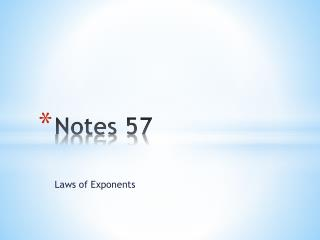DownloadDownload PresentationNotes 57

# Notes 57

Download Presentation## Notes 57

- - - - - - - - - - - - - - - - - - - - - - - - - - - E N D - - - - - - - - - - - - - - - - - - - - - - - - - - -
##### Presentation Transcript

1. Notes 57 Laws of Exponents

2. The factors of a power, such as 74, can be grouped in different ways. Notice the relationship of the exponents in each product. 7 • 7 • 7 • 7 = 74 (7 • 7 • 7) • 7 = 73 • 71 = 74 (7 • 7) • (7 • 7) = 72 • 72 = 74

3. A. 66 •63 6 6+ 3 6 9 n 5 + 7 n 12 Additional Example 1: Multiplying Powers with the Same Base Multiply. Write the product as one power. Add exponents. B. n5 •n7 Add exponents.

4. A. 66 •63 6 6+ 3 6 9 n 5 + 7 n 12 Additional Example 1: Multiplying Powers with the Same Base Multiply. Write the product as one power. Add exponents. B. n5 •n7 Add exponents.

5. A. 42 •44 Check It Out: Example 1 Multiply. Write the product as one power. B. x4 •x2

6. Check It Out: Example 1 Continued Multiply. Write the product as one power. C. 15• 152 D. p2• p2

7. 5555 5 5555 5 5 5 = = = 5 5 = 52 555 555 53 Notice what occurs when you divide powers with the same base.

8. 5 7 3 7 5 – 3 7 2 7 10 x 9 x 10 – 9 x Think: x = x 1 Additional Example 2: Dividing Powers with the Same Base Divide. Write the quotient as one power. A. Subtract exponents. B. Subtract exponents. x

9. Check It Out: Example 2 Divide. Write the quotient as one power. 9 9 A. 9 2 n 8 B. n 5

10. Additional Example 3: Raising a Power to a Power Simplify. A. (54)2 (54)2 54 • 2 Multiply exponents. 58 B. (67)9 (67)9 Multiply exponents. 67 • 9 663

11. 12 –3 2 3 12 • –3 2 3 Additional Example 3: Raising a Power to a Power Simplify. D. (172)–20 C. (172)–20 Multiply exponents. Multiply exponents. 172 • –20 17–40

12. Check It Out: Example 3 Simplify. A. (72)–1 B. (7–1)2

13. 4 –2 1 5 Check It Out: Example 3 Continued Simplify. D. (5–2)–3 C.

14. Additional Example 4: Application The speed of sound at sea level is 3.4029 x 102 meters per second. A ship that is 5 kilometers offshore sounds its horn. About how many seconds will pass before a person standing on shore will hear the sound? Write your answer in scientific notation.

15. Additional Example 4 Continued distance = rate x time 5 km = (3.4029 x 102) x t Write 5 km as meters. 5000 = (3.4029 x 102) x t 5 x 103 = (3.4029 x 102) x t Write 5000 in scientific notation. 3.4029 x 102 3.4029 x 102 5 103 1.469 x 101  t x = t 3.4029 x 102 102 It would take about 1.5 x 101 seconds for the sound to reach the shore.

16. Check It Out: Example 4 The diameter of a red blood cell is about 7.6 × 10-4 millimeters. Thai has a slide with a 2-cm drop of red blood cells on it. Approximately how many cells are on the slide? Write your answer in scientific notation. (Hint: Find the ratio of the size of the drop, in millimeters, to the size of one cell.)